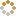﻿ N-B-U-T Online Judge :: Good Bye 2015 (One University One Question) Round III :: Ancient battle tree
• ### [D] Ancient battle tree

• 时间限制: 1000 ms　内存限制: 262144 K
• 问题描述
• There is a tree with n nodes and all the weight of edge is 1. So what’s the number of farthest distance point pairs.

• 输入
• There is less than 30 test cases.
For each test case, n (1 <= n <= 10000) is given.
Next n - 1 lines contain two integers u and v (1 <= u, v <= n) denoting an edge.
• 输出
• For each test case, output an interger result
• 样例输入
• ```5
1 2
2 3
3 4
4 5```
• 样例输出
• `1`
• 提示
• `For the sample input, there is only one farthest distance point pairs (node 1 and node 5), the distance is 4.`
• 来源
• `本站或者转载`
• 操作
﻿